# Ampere's Law

In electromagnetism, Ampère’s circuital law relates the integrated magnetic field around a closed loop to the electric current passing through the loop. In this article, let us learn in detail about Ampere’s Law.

## Who Was André-Marie Ampère?

André-Marie Ampère was a scientist who performed experiments with forces that act on current-carrying wires. The experiment was done in the late 1820s, around the same time when Faraday was working on his Faraday’s Law. Faraday and Ampere had no idea that their work would be combined by Maxwell Himself four years later.

## What Is Ampere’s Law?

According to Ampere’s law, magnetic fields are related to the electric current produced in them. The law specifies the magnetic field that is associated with a given current or vice-versa, provided that the electric field doesn’t change with time.

Ampere’s Law can be stated as:

“The magnetic field created by an electric current is proportional to the size of that electric current with a constant of proportionality equal to the permeability of free space.”

The equation explaining Ampere’s law which is the final Maxwell’s equation  is given below: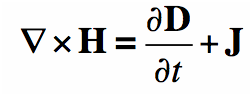Maxwell’s Equation

### What Is Ampere’s Circuital Law?

Ampere’s circuital law can be written as the line integral of the magnetic field surrounding closed-loop equals the number of times the algebraic sum of currents passing through the loop.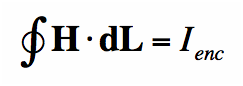Suppose a conductor carries a current I, then this current flow generates a magnetic field that surrounds the wire.

The equation’s left side describes that if an imaginary path encircles the wire and the magnetic field is added at every point, then it is numerically equal to the current encircled by this route, indicated by Ienc.

## To Know in Detail about Ampere’s Circuital Law, See the below VideoYou may also want to check out these topics given below!

### Determining Magnetic Field by Ampere’s Law (Example)

Suppose you have a long enough wire that carries a constant current I in amps. How would you determine the magnetic field wrapping the wire at any distance r from the wire?

In the figure below ( Figure 1), a long wire exists that carries current in Amps. We need to find out how much is the magnetic field at a distance r. Therefore, we sketch an imaginary route around the wire indicated by dotted blue toward the right in the figure.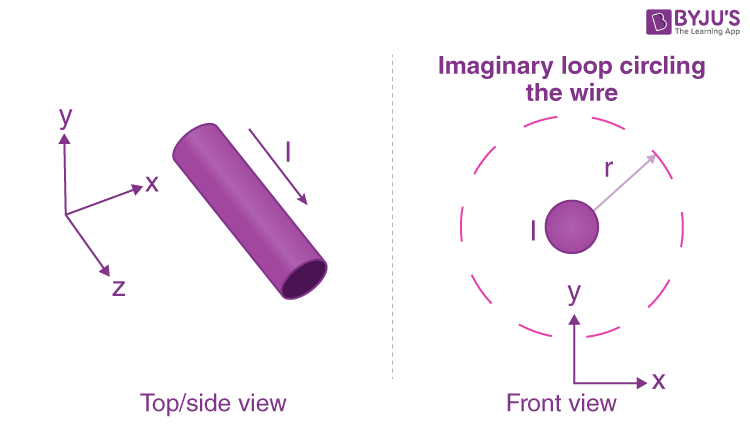Figure 1

According to the second equation, if the magnetic field is integrated along the blue path, then it has to be equal to the current enclosed, I.

The magnetic field doesn’t vary at a distance r due to symmetry. The path length (in blue) in figure 1 is equal to the circumference of a circle,2πr.

When a constant value H is added to the magnetic field, the equation’s left side looks like this: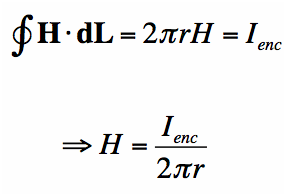We have figured the magnitude of the field H. Since r is arbitrary, the value of the field H is known.

The magnetic field lowers in magnitude as we move wider according to the equation. Hence, Ampere’s law can be applied to calculate the extent of the magnetic field surrounding the wire. The field H is a vector field that reveals that each region has a direction and a magnitude. The field’s direction is tangential at every point to the imaginary loops, as shown in figure 2, and the right-hand rule finds the direction of the magnetic field.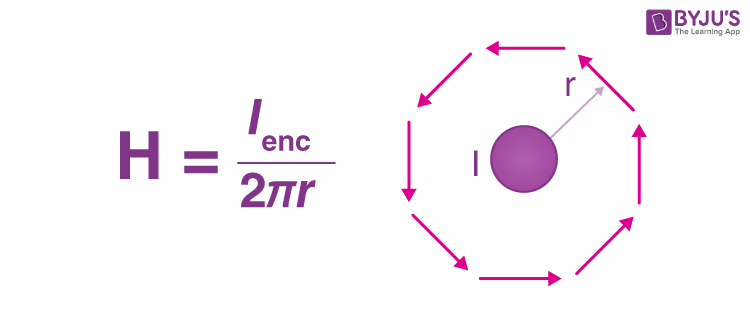### Applications of Ampere’s Law

Ampere’s Law is used to :

• Determine the magnetic induction due to a long current-carrying wire.
• Determine the magnetic field inside a toroid.
• Determine the magnetic field created by a long current-carrying conducting cylinder.
• Determine the magnetic field inside the conductor.
• Find forces between currents
• ## To Learn about Ampere’s Law, Moving Charges and Magnetism, Click on the Video Below## Frequently Asked Questions – FAQs

Q1

### State Ampere’s law.

Ampere’s law states that “The magnetic field created by an electric current is proportional to the size of that electric current with a constant of proportionality equal to the permeability of free space.”
Q2

### Name the scientist who performed experiments with forces that act on current-carrying wires?

André-Marie Ampère.
Q3

True.
Q4

True.
Q5

### State Ampere’s circuital law.

Ampere’s circuital law states that “the line integral of the magnetic field surrounding closed-loop equals to the number of times the algebraic sum of currents passing through the loop.”

## Watch the Video and Learn about Magnetic Field Due to Solenoid and Properties of Magnetic Field LinesTo know more about real-time applications of Ampere’s law along with Gauss law and magnetic field due to Solenoid, visit BYJU’S – The learning app.

Test your knowledge on Ampere's law